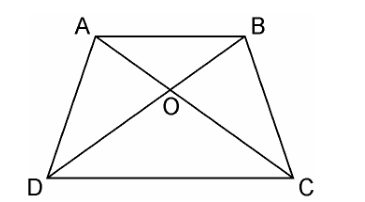# Diagonals AC and BD of a trapezium ABCD with AB || DC intersect each other at the point O. Using a similarity criterion for two triangles, show that AO/OC = OB/OD

Given that

Diagonals AC and BD of a trapezium ABCD with AB || DC intersect each other at the point O

To Prove

AO/OC = OB/ODProof

In ΔDOC and ΔBOA,

Since, AB || CD, therefore the alternate interior angles are equal,

That is, ∠CDO = ∠ABO ….(i)

And,

∠DCO = ∠BAO …(ii)

Also, vertically opposite angles are equal;

That is,∠DOC = ∠BOA…(iii)

Hence, by AAA similarity criterion,

ΔDOC ~ ΔBOA [From eq(i) , (ii) and (iii)]

Since the triangles are similar, therefore the corresponding sides are proportional.

DO/BO = OC/OA

⇒OA/OC = OB/OD

Hence, proved.